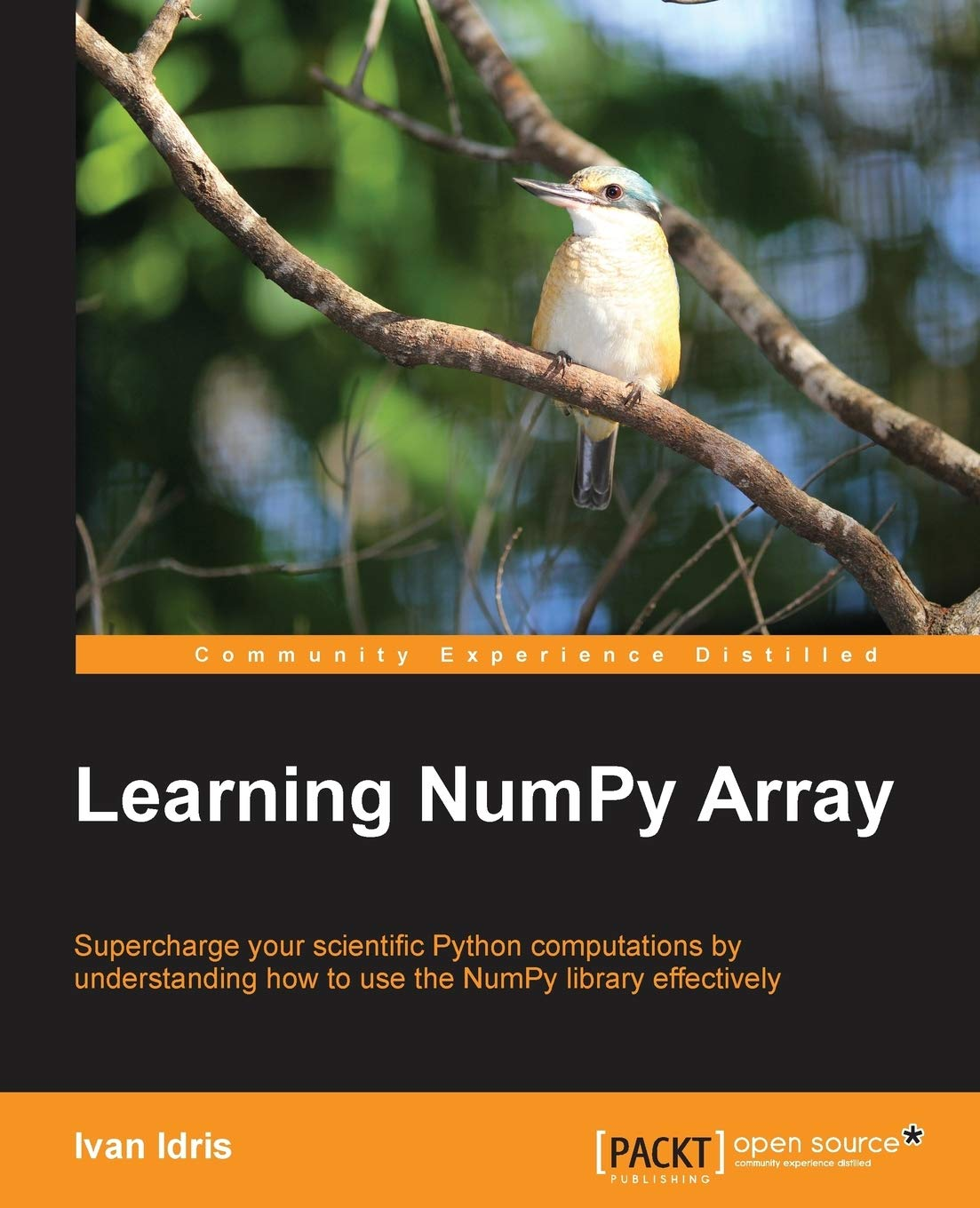### Learning NumPy Array

Posted by jack_miller | Published a year agoWith 9 ratings

#### Purchased At: \$26.99

Supercharge your scientific Python computations by understanding how to use the NumPy library effectively

• Improve the performance of calculations with clean and efficient NumPy code
• Analyze large data sets using statistical functions and execute complex linear algebra and mathematical computations
• Perform complex array operations in a simple manner

## Who This Book Is For

This book is for programmers, scientists, or engineers, who have basic Python knowledge and would like to be able to do numerical computations with Python.

## What You Will Learn

• Install NumPy and discover its arrays and features
• Perform data analysis and complex array operations with NumPy
• Analyze time series and perform signal processing
• Understand NumPy modules and explore the scientific Python ecosystem
• Improve the performance of calculations with clean and efficient NumPy code
• Analyze large data sets using statistical functions and execute complex linear algebra and mathematical computations

## In Detail

NumPy is an extension of Python, which provides highly optimized arrays and numerical operations. NumPy replaces a lot of the functionality of Matlab and Mathematica specifically vectorized operations, but in contrast to those products is free and open source. In today's world of science and technology, it is all about speed and flexibility.

This book will teach you about NumPy, a leading scientific computing library. This book enables you to write readable, efficient, and fast code, which is closely associated to the language of mathematics. Save thousands of dollars on expensive software, while keeping all the flexibility and power of your favorite programming language.

You will learn about installing and using NumPy and related concepts. At the end of the book we will explore related scientific computing projects. This book will give you a solid foundation in NumPy arrays and universal functions. Learning NumPy Array will help you be productive with NumPy and write clean and fast code.